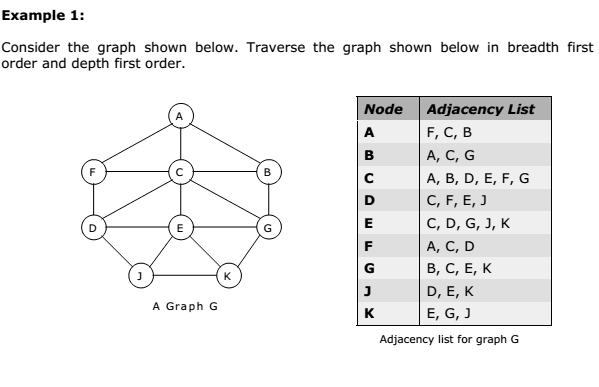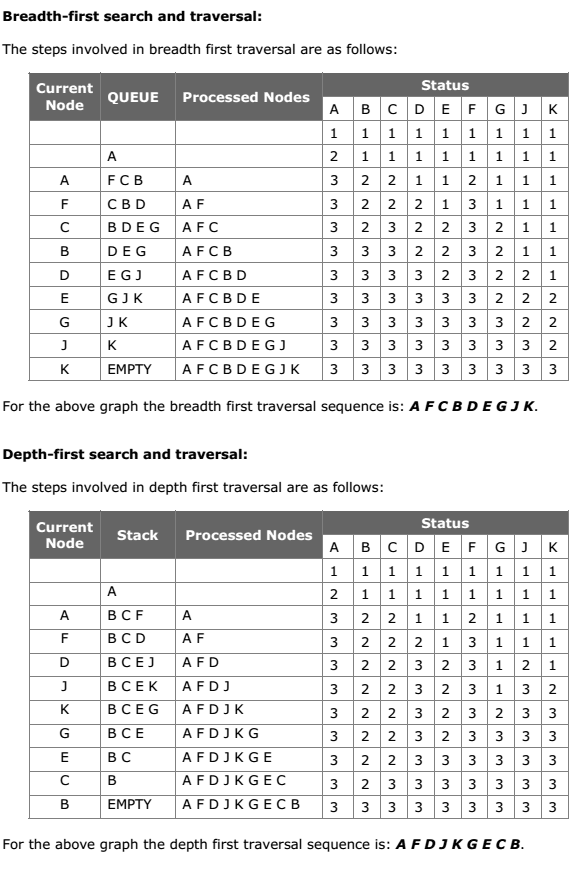Many graph algorithms require one to systematically examine the nodes and edges of a graph G. There are two standard ways to do this. They are:

• Breadth first traversal (BFT)
• Depth first traversal (DFT)

The BFT will use a queue as an auxiliary structure to hold nodes for future processing and the DFT will use a STACK.

During the execution of these algorithms, each node N of G will be in one of three states, called the status of N, as follows:

1. STATUS = 1 (Ready state): The initial state of the node N.
2. STATUS = 2 (Waiting state): The node N is on the QUEUE or STACK, waiting to be processed.
3. STATUS = 3 (Processed state): The node N has been processed.

Both BFS and DFS impose a tree (the BFS/DFS tree) on the structure of graph. So, we can compute a spanning tree in a graph. The computed spanning tree is not a minimum spanning tree. The spanning trees obtained using depth first search are called depth first spanning trees. The spanning trees obtained using breadth first search are called Breadth first spanning trees.

Breadth first search and traversal:

The general idea behind a breadth first traversal beginning at a starting node A is as follows. First we examine the starting node A. Then we examine all the neighbors of A. Then we examine all the neighbors of neighbors of A. And so on. We need to keep track of the neighbors of a node, and we need to guarantee that no node is processed more than once. This is accomplished by using a QUEUE to hold nodes that are waiting to be processed, and by using a field STATUS that tells us the current status of any node. The spanning trees obtained using BFS are called

Breadth first spanning trees.

Breadth first traversal algorithm on graph G is as follows:

This algorithm executes a BFT on graph G beginning at a starting node A.

Initialize all nodes to the ready state (STATUS = 1).

1. Put the starting node A in QUEUE and change its status to the waiting state (STATUS = 2).
2. Repeat the following steps until QUEUE is empty:a. Remove the front node N of QUEUE. Process N and change the status of N to the processed state (STATUS = 3).
b. Add to the rear of QUEUE all the neighbors of N that are in the ready state (STATUS = 1), and change their status to the waiting state (STATUS = 2).
3. Exit.

Depth first search and traversal:

Depth first search of undirected graph proceeds as follows: First we examine the starting node V. Next an unvisited vertex ‘W’ adjacent to ‘V’ is selected and a depth first search from ‘W’ is initiated. When a vertex ‘U’ is reached such that all its adjacent vertices have been visited, we back up to the last vertex visited, which has an unvisited vertex ‘W’ adjacent to it and initiate a depth first search from W. The search terminates when no unvisited vertex can be reached from any of the visited ones.

This algorithm is similar to the inorder traversal of binary tree. DFT algorithm is similar to BFT except now use a STACK instead of the QUEUE. Again field STATUS is used to tell us the current status of a node.

The algorithm for depth first traversal on a graph G is as follows.

This algorithm executes a DFT on graph G beginning at a starting node A.

1.  Initialize all nodes to the ready state (STATUS = 1).
2. Push the starting node A into STACK and change its status to the waiting state (STATUS = 2).
3. Repeat the following steps until STACK is empty:
a. Pop the top node N from STACK. Process N and change the status of N to the processed state (STATUS = 3).
b. Push all the neighbors of N that are in the ready state (STATUS = 1), and change their status to the waiting state (STATUS = 2).
4. Exit.You may be interested in:
Data Structures and Algorithms – MCQs.
Data Structures and Algorithms Online Tests